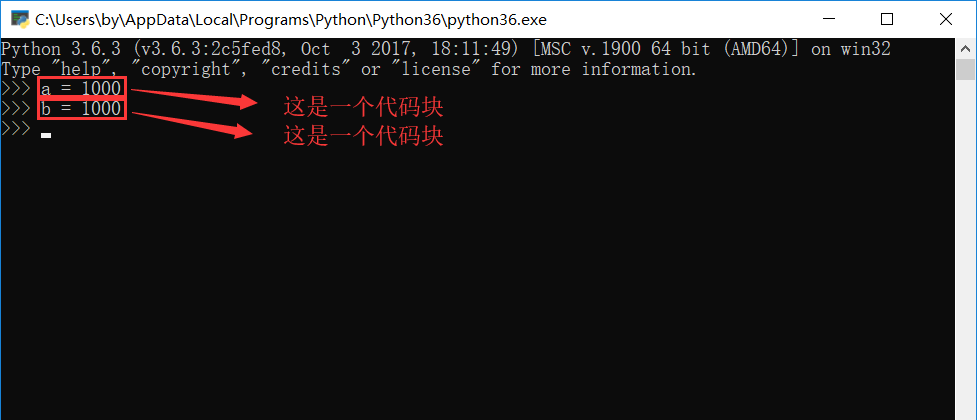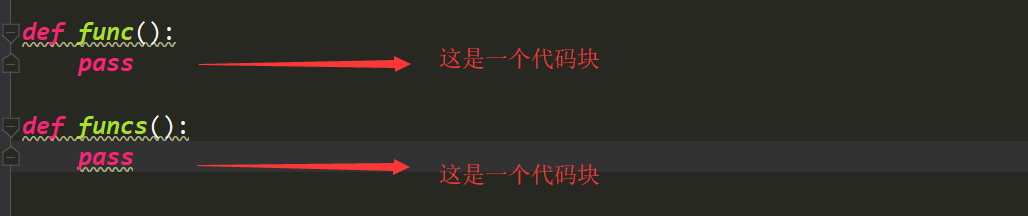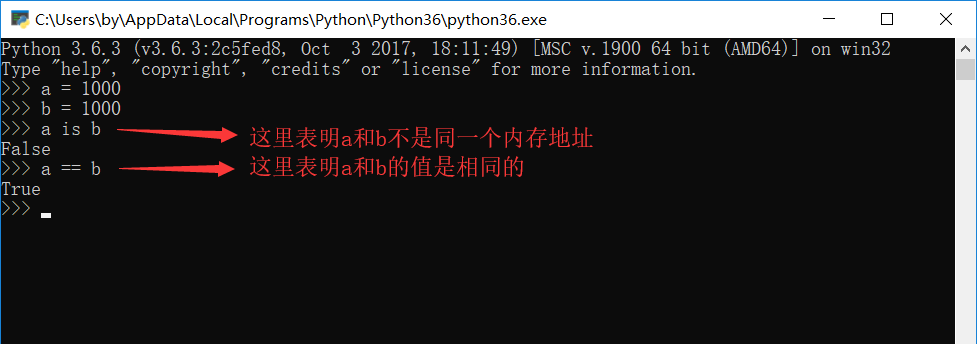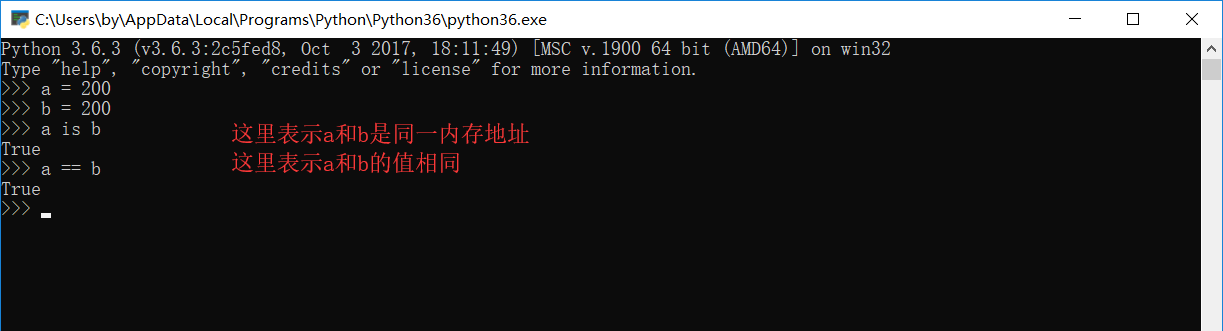# 万恶之源 – Python基础数据类型三

By | 2018年10月21日

### 字典

字典的简单介绍

字典(dict)是python中唯?的?个映射类型.他是以{ }括起来的键值对组成.

在dict中key是 唯?的.在保存的时候, 根据key来计算出?个内存地址. 然后将key-value保存在这个地址中.

这种算法被称为hash算法, 所以, 切记, 在dict中存储的key-value中的key必须是可hash的, 如果你搞不懂什么是可哈希, 暂时可以这样记,

可以改变的都是不可哈希的, 那么可哈希就意味着不可变. 这个是为了能准确的计算内存地址?规定的.

已知的可哈希(不可变)的数据类型: int, str, tuple, bool 不可哈希(可变)的数据类型: list, dict, set

语法:{‘key1′:1,’key2’:2}

注意: key必须是不可变(可哈希)的. value没有要求.可以保存任意类型的数据

```# 合法
dic = {123: 456, True: 999, "id": 1, "name": 'sylar', "age": 18, "stu": ['帅

print(dic)
print(dic[True])
print(dic['id'])
print(dic['stu'])
print(dic[(1, 2, 3)])

# 不合法
# dic = {[1, 2, 3]: '周杰伦'} # list是可变的. 不能作为key
# dic = {{1: 2}: "哈哈哈"} # dict是可变的. 不能作为key
dic = {{1, 2, 3}: '呵呵呵'} # set是可变的, 不能作为key```

```dic = {}

dic['name'] = '汪峰'
dic['age'] = 18
print(dic)

{'name': '汪峰', 'age': 18}

# 如果dict中没有出现这个key,就会将key-value组合添加到这个字典中

# 如果dict中没有出现过这个key-value. 可以通过setdefault设置默认值

s1 = dic.setdefault('王菲')
print(s1)
print(dic)

None
# 返回的是添加进去的值
{'王菲': None}
# 我们使用setdefault这个方法 里边放的这个内容是我们字典的健,这样我们添加出来的结果

dic.setdefault('王菲',歌手)
# 这样就是不会进行添加操作了,因为王菲在dic这个字典中存在
# 总结: 当setdefault中第一个参数存在这个字典中就就不进行添加操作,否则就添加

dic1 = {}
s2 = dic1.setdefault('王菲','歌手')
print(s2)
print(dic1)

{'王菲': '歌手'}```

```dic = {'剑圣':'易','哈啥给':'剑豪','大宝剑':'盖伦'}

s = dic.pop('哈啥给')   # pop删除有返回值,返回的是被删的值
print(s)

print(dic)    # 打印删除后的字典

dic.popitem()  # 随机删除  python3.6是删除最后一个
print(dic)

dic.clear()  # 清空```

```dic = {'剑圣':'易','哈啥给':'剑豪','大宝剑':'盖伦'}

dic['哈啥给'] = '剑姬'   # 当哈啥给是字典中的健这样写就是修改对应的值,如果不存在就是添加

print(dic)

dic.update({'key':'v','哈啥给':'剑姬'})

# 当update中的字典里没有dic中键值对就添加到dic字典中,如果有就修改里边的对应的值
print(dic)```

```dic = {'剑圣':'易','哈啥给':'剑豪','大宝剑':'盖伦'}

s = dic['大宝剑']        #通过健来查看,如果这个健不在这个字典中.就会报错
print(s)

s1 = dic.get('剑圣')     #通过健来查看,如果这个健不在这个字典中.就会返回None
print(s1)

s2 = dic.get('剑姬','没有还查你是不是傻')  # 我们可以在get查找的时候自己定义返回的结果
print(s2)```

```dic = {'k1': "v1", "k2": "v2", "k3": [11,22,33]}

```key_list = dic.keys()
print(key_list)

dict_keys(['剑圣', '哈啥给', '大宝剑'])
# 一个高仿列表,存放的都是字典中的key

value_list = dic.values()
print(value_list)

dict_values(['易', '剑豪', '盖伦'])
#一个高仿列表,存放都是字典中的value

key_value_list = dic.items()
print(key_value_list)

dict_items([('剑圣', '易'), ('哈啥给', '剑豪'), ('大宝剑', '盖伦')])

# 一个高仿列表,存放是多个元祖,元祖中第一个是字典中的键,第二个是字典中的值　　``````dic = {'剑圣':'易','哈啥给':'剑豪','大宝剑':'盖伦'}

for i in dic:
print(i)

for i in dic.keys():
print(i)```dic = {'剑圣':'易','哈啥给':'剑豪','大宝剑':'盖伦'}
for i in dic:
print(dic[i])

for i in dic.values():
print(i)```dic = {'剑圣':'易','哈啥给':'剑豪','大宝剑':'盖伦'}
for i in dic.items():
print(i)

('剑圣', '易')
('哈啥给', '剑豪')
('大宝剑', '盖伦')```

```a,b = 1,2
print(a,b)

1 2

a,b = ('你好','世界')
print(a,b)

a,b = ['你好','大飞哥']
print(a,b)

a,b = {'汪峰':'北京北京','王菲':'天后'}
print(a,b)

```for k,v in dic.items():
print('这是键',k)
print('这是值',v)

### 字典的嵌套

```dic = {
'name':'汪峰',
'age':48,
'wife':[{'name':'国际章','age':38}],
'children':['第一个熊孩子','第二个熊孩子']
}
```

```d1 = dic['wife']['name']
print(d1)
```

```d2 = dic['children']
print(d2)```

```d3 = dic['children']
print(d3)```

```dic1 = {
'name':['alex',2,3,5],
'job':'teacher',
'oldboy':{'alex':['python1','python2',100]}
}
1，将name对应的列表追加?个元素’wusir’。
2，将name对应的列表中的alex?字??写。
3，oldboy对应的字典加?个键值对’?男孩’,’linux’。
4，将oldboy对应的字典中的alex对应的列表中的python2删除```

### 小数据池

```根据提示我们从官方文档找到了这样的说法：
A Python program is constructed from code blocks. A block is a piece of Python program text that is executed as a unit. The following are blocks: a module, a function body, and a class definition. Each command typed interactively is a block. A script file (a file given as standard input to the interpreter or specified as a command line argument to the interpreter) is a code block. A script command (a command specified on the interpreter command line with the ‘-c‘ option) is a code block. The string argument passed to the built-in functions eval() and exec() is a code block.
A code block is executed in an execution frame. A frame contains some administrative information (used for debugging) and determines where and how execution continues after the code block’s execution has completed.```

Python程序是由代码块构造的。块是一个python程序的文本，他是作为一个单元执行的。OK，那么现在我们了解了代码块，我们就来看看小数据池和代码块有啥关系,

### id is ==

```name = 'meet'
s_id = id(name)   # 通过内置方法获取name变量对应的值在内存中的编号
print(s_id)       # 2055782908568 这就是name在内存中的编号
```

== 是比较的两边的数值是否相等，而 is 是比较的两边的内存地址是否相等。 如果内存地址相等，那么这两边其实是指向同一个内存地址。```对于整数，Python官方文档中这么说：
The current implementation keeps an array of integer objects for all integers between -5 and 256, when you create an int in that range you actually just get back a reference to the existing object. So it should be possible to change the value of 1. I suspect the behaviour of Python in this case is undefined.

Incomputer science, string interning is a method of storing only onecopy of each distinct string value, which must be immutable. Interning strings makes some stringprocessing tasks more time- or space-efficient at the cost of requiring moretime when the string is created or interned. The distinct values are stored ina string intern pool. –引自维基百科```

在python中对-5到256之间的整数会被驻留在内存中. 将?定规则的字符串缓存. 在使? 的时候, 内存中只会创建?个该数据的对象. 保存在?数据池中. 当使?的时候直接从?数据 池中获取对象的内存引?. ?不需要创建?个新的数据. 这样会节省更多的内存区域.

能够提??些字符串, 整数的处理速度. 省略的创建对象的过程.

在’池’中创建或者插入新的内容会花费更多的时间.

-5~256是会被加到?数据池中的. 每次使?都是同?个对象.

1. 如果字符串的?度是0或者1, 都会默认进?缓存

2. 字符串?度?于1, 但是字符串中只包含字?, 数字, 下划线时才会缓存

3. ?乘法的到的字符串.

①. 乘数为1, 仅包含数字, 字?, 下划线时会被缓存. 如果 包含其他字符, ??度<=1 也会被驻存

②. 乘数?于1 . 仅包含数字, 字?, 下划 线这个时候会被缓存. 但字符串?度不能?于20 4. 指定驻留. 我们可以通过sys模块中的intern()函数来指定要驻留的内容.

OK. 到?前为?. 我们已经了解了python的?数据池的?些基本情况了. 但是!!!!### 集合(set)

set集合是python的?个基本数据类型. ?般不是很常?. set中的元素是不重复的.?序的.? ?的元素必须是可hash的(int, str, tuple,bool), 我们可以这样来记. set就是dict类型的数据但 是不保存value, 只保存key. set也?{}表?

```set1 = {'1','alex',2,True,[1,2,3]} # 报错
set2 = {'1','alex',2,True,{1:2}} # 报错
set3 = {'1','alex',2,True,(1,2,[2,3,4])} # 报错
```

set中的元素是不重复的, 且?序的.

```s = {"周杰伦", "周杰伦", "周星星"}
print(s)

{'周星星', '周杰伦'}
```

```# 给list去重复
lst = [45, 5, "哈哈", 45, '哈哈', 50]
lst = list(set(lst)) # 把list转换成set, 然后再转换回list
print(lst)
```

set集合增删改查

```s = {"刘嘉玲", '关之琳', "王祖贤"}
print(s)
print(s)
s = {"刘嘉玲", '关之琳', "王祖贤"}
s.update("麻花藤") # 迭代更新
print(s)
s.update(["张曼?", "李若彤","李若彤"])
print(s)
```

```s = {"刘嘉玲", '关之琳', "王祖贤","张曼?", "李若彤"}
item = s.pop() # 随机弹出?个.
print(s)
print(item)
s.remove("关之琳") # 直接删除元素
# s.remove("??疼") # 不存在这个元素. 删除会报错
print(s)
s.clear() # 清空set集合.需要注意的是set集合如果是空的. 打印出来是set() 因为要和
dict区分的.
print(s) # set()
```

```# set集合中的数据没有索引. 也没有办法去定位?个元素. 所以没有办法进?直接修改.
# 我们可以采?先删除后添加的?式来完成修改操作
s = {"刘嘉玲", '关之琳', "王祖贤","张曼?", "李若彤"}
# 把刘嘉玲改成赵本?
s.remove("刘嘉玲")
print(s)
```

```# set是?个可迭代对象. 所以可以进?for循环
for el in s:
print(el)
```

```s1 = {"刘能", "赵四", "???"}
s2 = {"刘科?", "冯乡?", "???"}
# 交集
# 两个集合中的共有元素
print(s1 & s2) # {'???'}
print(s1.intersection(s2)) # {'???'}
# 并集
print(s1 | s2) # {'刘科?', '冯乡?', '赵四', '???', '刘能'}
print(s1.union(s2)) # {'刘科?', '冯乡?', '赵四', '???', '刘能'}
# 差集
print(s1 - s2) # {'赵四', '刘能'} 得到第?个中单独存在的
print(s1.difference(s2)) # {'赵四', '刘能'}
# 反交集
print(s1 ^ s2) # 两个集合中单独存在的数据 {'冯乡?', '刘能', '刘科?', '赵四'}
print(s1.symmetric_difference(s2)) # {'冯乡?', '刘能', '刘科?', '赵四'}
s1 = {"刘能", "赵四"}
s2 = {"刘能", "赵四", "???"}
# ?集
print(s1 < s2) # set1是set2的?集吗? True
print(s1.issubset(s2))
# 超集
print(s1 > s2) # set1是set2的超集吗? False
print(s1.issuperset(s2))
```

set集合本?是可以发?改变的. 是不可hash的. 我们可以使?frozenset来保存数据. frozenset是不可变的. 也就是?个可哈希的数据类型

```s = frozenset(["赵本?", "刘能", "???", "?跪"])
dic = {s:'123'} # 可以正常使?了
print(dic)
```

via:https://www.cnblogs.com/guobaoyuan/p/9824286.html